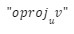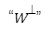Online Math Center

# Math Symbols and Their Meanings Part VIII: Linear and Multilinear Algebra

We are approaching the end of our Math Symbols and Their Meanings series, but until then, the OMC math tutors will help you remember the fundamentals of the mathematical language with linear and multilinear algebra.

### The Basics Of Linear Algebra

Did you know that linear algebra is the foundation of machine learning? It is used primarily in statistics and data mathematics. Linear algebra symbols include operators used in vector, matrix and space-related functions.

#### Linear Algebra Symbols – Vectors and space vectors

“ -v “

The symbol above indicates the additive inverse property of vector v, meaning the opposite value of vector v.

kv “

Scalar product of vector v by scalar k. The scalar product is a multiplication method of two vectors by taking one vector in the direction of the other and multiplying it times the magnitude of the other vector. This is a basic vector operation that you should master if you are interested in astrophysics.

“ u + v ”  or  “ u – v ”

The simplest operations in linear algebra, these symbols indicate the sum and difference operations between two vectors.

“ u · x “

Similar to scalar product, the dot product of vectors, a multiplication method, takes two equal-length sequences of numbers (usually coordinate vectors) and returns a single number.

“ u x v ”

This operation indicates the cross product of vectors u and v.  The cross product refers to a third vector, which is perpendicular to the two original vectors.

“ u ⌃ v “

This operation symbol indicates the wedge product of vectors u and v. The wedge product of vectors is used in geometry to study areas, volumes, and exterior spaces. The wedge product is actually the multiplication operation in exterior algebra.

“ ⟨u, v⟩ “

These brackets represent the inner product of vectors, meaning the generalization of the dot product.

“ u ⦻ v “

This is the indication of the outer product of vectors. The outer product is a tensor product of two coordinate vectors, resulting in a matrix.

“ ‖v‖ “

The symbol above indicates the norm of vector v, meaning the length or magnitude of the vector.

” ‖v‖p

This indicates the p-norm of vector v.

“ v ”

This symbol indicates the unit vector in the direction of v.This symbol indicates the projection of vector v onto vector u.This symbol indicates the orthogonal projection of vector v onto vector u.

“ker(f)”

This symbol indicates the Kernel of the linear map (f). Also referred to as the null-space, it denotes the set of all vectors in the input space, mapped under the linear transformation to the null vector of the output space.

“span(S)”

It indicates the span of a set of vectors S, meaning the set of all linear combinations of vectors (S).

“dim(V)”

The symbol above indicates the dimension of vector space V.

“W1 + W2

It indicates the sum of vectors W1 and W2. Sum refers to vectors.

“W1W2”

It indicates the direct sum of subspaces W1 and W2. Direct sum refers to subspaces.

“V1 x V2

This operation indicates the direct product of vector spaces V1 and V2

“V1V2”

This operation indicates the tensor product of vector spaces V1 and V2

“ V/ W “

This symbol indicates the quotient space of vector V over subspace vector W.

“ L(V1, V2) “

It symbolizes the set of linear maps from vector space V1 to vector space V2It indicates the orthogonal complement of subspace W.

“ V* “

This symbol indicates the dual space of vector space v.### Linear Algebra Symbols – Matrix

The matrix symbols are similar to vector symbols mentioned above, here are some of them:“-A” – the additive inverse of matrix A;

• “ ka “ – refers to the scalar product of matrix A by scalar k;

• “ A + B “ and “A – B” – indicates the sum and difference operations of matrices A and B

• “ AB “ – indicates the product of matrices A and B;

• “ A ￮ B “ or “ A ⌾ B “ – indicates the Hadamard product of matrices A and B, it is a binary operation that takes two matrices of the same dimensions and produces another matrix of the same dimension as the operands;

• “ A ⦻ B “ – indicates the Kronecker product of matrices A and B;

• “ AT” – indicates the transpose of matrix A;

• “ AH” or “ A* “ – indicates the conjugate transpose of matrix A;

• “ A-1” – indicates the multiplicative inverse of matrix A;

• “ tr(A)” – indicates the trace of matrix A;

• “ det(A) “ or “|A| “ – indicates the determinant of matrix A;

• “ ‖A‖ “ – indicates the norm of matrix A;

• ” ‖A‖p ” – indicates the P-norm of matrix A;

• “ rank(A)” – indicates the rank of matrix A;

### The Basic Symbols Of Multilinear Algebra

The Levi-Civita symbol is a tensor of rank 3 and meets the following conditions:

= 0, if any two values are the same;

= -1, if i, j, k are an odd permutation of 1, 2, 3

= 1, if i, j, k are an even permutation of 1, 2, 3This symbol is widely known as Einstein’s notation, or Einstein’s summation convention/notation.

“ Sym(V) ”

This symbol refers to symmetric algebra, the commutative algebra over the field K that contains V.

Linear and Multilinear algebra are complex mathematical operations used in spatial geometry and physics or astronomy. For passionate mathematicians, linear and multilinear algebra is fundamental.

### Online Math Center Can Improve Your Math Skills

At the Online Math Center, we make sure to provide high-quality resources for middle and high-school students.

Our math tutoring lessons are tailored according to the students’ needs, be it individual tutoring or math competition practice.

Contact us any time for more details on our math tutoring curriculum!

## Step One

It only takes two steps to schedule a free lesson with an OMC representative.

To get started, please tell us who you are:

## Dear Parent!

Thank you for placing your trust in us to educate your child!

Congratulations on joining the Online Math Center!
See you in class.

## Thank you!

Our manager will be in contact with you shortly.

## Step Two

Let us know how to contact you. One of our representatives will get back to you shortly.

## Step Two

Awesome! We need to get in touch with your parent or guardian for further discussion. Please check in with them before filling out the form below with their information.

## Step One

It only takes two steps to schedule an appointment with an OMC representative.

To get started, please tell us who you are: